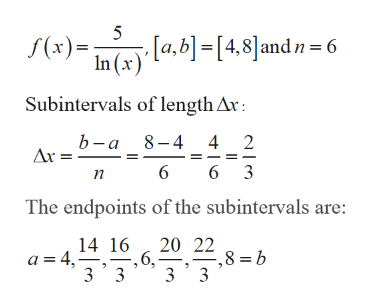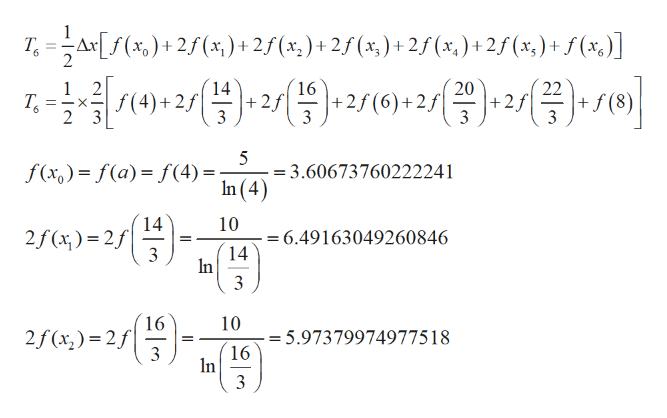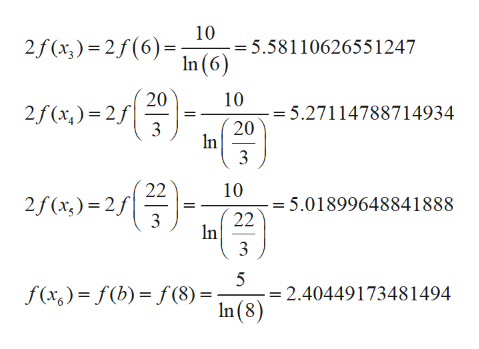# Use the trapezoidal rule and Simpson's rule to approximate the value of the definite integral. (Give your answers correct to 4 decimal places.) 4∫85/ln(x) dx; n=6

Question
1 views

Use the trapezoidal rule and Simpson's rule to approximate the value of the definite integral. (Give your answers correct to 4 decimal places.)

485/ln(x) dx; n=6

check_circle

Step 1

Given:help_outlineImage Transcriptionclose5 [a,b]=[4,8]andn= 6 In (x (x) Subintervals of length Ar: 8-4 4 b-a 2 Ar = 6 6 3 п The endpoints of the subintervals are: 20 22 8 b 14 16 ,6, 3 3 3 3 a=4, fullscreen
Step 2

Use the trapezoidal rule and Simpson's rule to approximate the value

Trapezoidal:help_outlineImage TranscriptioncloseT Ar(x) 2f (x,)+2f(x.)+2f(x,)+ 2f(x,)+2f(x,)+ f 22 16 +2f 3 4)222/(6) 20 1 Т. 2 2 +f (8) 3 + 2f (6)+2f +2f =-X- - 3 5 = 3.60673760222241 In (4 f(x) = f(a) f(4) 14 10 2f (x)2f 3 = 6.49163049260846 14 In 3 16 2f(x) 2f 10 = 5.97379974977518 16 In 3 fullscreen
Step 3

Step 2 as fo...help_outlineImage Transcriptionclose10 = 5.58110626551247 In (6) 2f (x;) = 2f(6)= 20 10 2f (x,) = 2f 3 = 5.27114788714934 20 In 3 22 10 2f (x,) = 2f 5.01899648841888 22 In 3 3 = 2.40449173481494 In(8) f(x,) = f(b) = f (8) = fullscreen

### Want to see the full answer?

See Solution

#### Want to see this answer and more?

Solutions are written by subject experts who are available 24/7. Questions are typically answered within 1 hour.*

See Solution
*Response times may vary by subject and question.
Tagged in

### Other